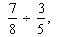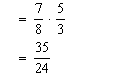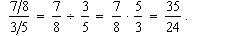How to divide two fractions, Mathematics

Assignment Help:

Q. How to divide two fractions?If you want to divide two fractions,You invert the second fraction (that means, turn it upside-down) and multiply (change the division to a multiplication):That's easy, right? Just be sure it's the second fraction you invert, and not the first!

Note: in Algebra and higher, we don't use the x sign any more. We just use fraction bars. Instead of writing 5 / 3, we write:

5/3

So, when you see something that looks like this:

(7/8)/(3/5)

It's really a division problem in disguise!Fractions like that, which have fractions inside them, are called compound fractions. (No, not "compound fractures"!!!) These compound fractions aren't mysterious. They're just divisions.

Elements of marketing, what is the elements of marketing

what is the elements of marketing

Ellipse, How we find locus of the middle points of chord of an ellipse whic...

How we find locus of the middle points of chord of an ellipse which are drawn through the positive end of the minor axes

Rhjuu, Ask questutfjion #Minimum 100 words accepted#

Ask questutfjion #Minimum 100 words accepted#

Area problem, Area Problem Now It is time to start second kind of inte...

Area Problem Now It is time to start second kind of integral: Definite Integrals.  The area problem is to definite integrals what tangent & rate of change problems are to d

Mean, Jocelyn has 6 birds, Their mean age is 10. The mode of their ages is ...

Jocelyn has 6 birds, Their mean age is 10. The mode of their ages is 8. What might their ages be? Show your work.

Ratio, 2qt :6qt::x :48? help me solve x

2qt :6qt::x :48? help me solve x

Evaluate the infinite limits of given limits, Evaluate following limits. ...

Evaluate following limits. Solution Therefore we will taking a look at a couple of one-sided limits in addition to the normal limit here. In all three cases notice

Describe visualize solutions of simultaneous equations, Describe Visualize ...

Describe Visualize Solutions of Simultaneous Equations ? By drawing the graph of each equation in a system of equations, you can see a picture of the system's solutions. Fo

Find out the greatest common factor, Find out the Greatest Common Factor? ...

Find out the Greatest Common Factor? The largest number that is a common factor of two numbers (that is, both numbers share the same factor) is called the greatest common facto